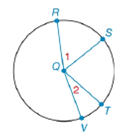Chapter 6.4, Problem 20EElementary Geometry For College St...

7th Edition
Alexander + 2 others
ISBN: 9781337614085

Solutions

Chapter
SectionElementary Geometry For College St...

7th Edition
Alexander + 2 others
ISBN: 9781337614085
Textbook Problem

a) If m R S ⌢ > m T V ⌢ , write an inequality that compares m ∠ 1 with m ∠ 2 .b) If m ∠ 1 > m ∠ 2 , write and inequality that compares m R S ⌢ with m T V ⌢ .To determine

(a)

To write:

An inequality that compares m1 with m2.

Explanation

Given:

mRS>mTV and the figure given below

Theorem:

In a circle (or in congruent circles) containing two unequal arcs, the larger arc corresponds to the larger central angle.

Calculation:

From the theorem,

Since the arcs are unequal i.e. mRS>mTV and from the given figure,

To determine

(b)

To write:

An inequality that compares mRS with mTV.

Still sussing out bartleby?

Check out a sample textbook solution.

See a sample solution

The Solution to Your Study Problems

Bartleby provides explanations to thousands of textbook problems written by our experts, many with advanced degrees!

Get Started

Find more solutions based on key concepts